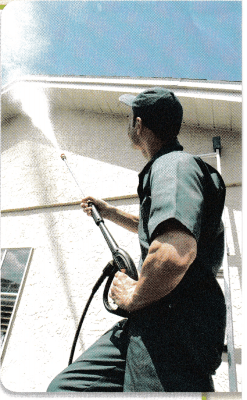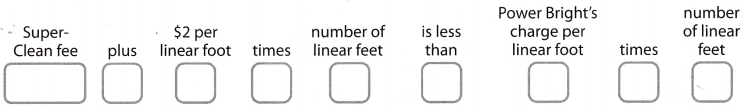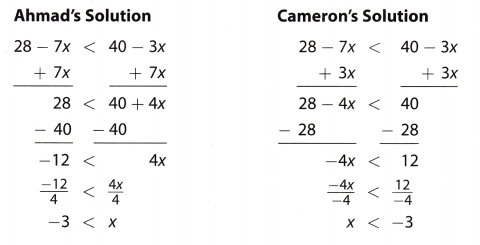# Texas Go Math Grade 8 Lesson 11.3 Answer Key Inequalities with the Variable on Both Sides

Refer to our Texas Go Math Grade 8 Answer Key Pdf to score good marks in the exams. Test yourself by practicing the problems from Texas Go Math Grade 8 Lesson 11.3 Answer Key Inequalities with the Variable on Both Sides.

## Texas Go Math Grade 8 Lesson 11.3 Answer Key Inequalities with the Variable on Both Sides

Modeling a Real-World Situation with an inequality

Many real-world situations can be modeled by inequalities. Some phrases that indicate an inequality are “less than 7” “greater than7” no more than,” and “at least.”Super-Clean house cleaning company charges a fee of $384 to power wash a house plus$2 per linear foot. Power Bright charges $6 per linear foot, but no flat fee. Write an inequality that can be solved to find the number of linear feet a house must have to make the total cost charged by Super-Clean less than the cost charged by Power Bright. A. Translate from words into symbols. Let! represent the number of ______________ of the house.Answer: Less than 7 symbol = <7. Greater than 7 symbol = >7 No more than symbol = >/ 7 At least 7 = > or = 7 Given that, Super-Clean house cleaning company charges a fee to power wash a house =$384 + $2 per linear foot. Power Bright charges$6 per linear foot, but no flat fee.
Let us consider the number of linear feet of houses = x
$384 +$2x < $6xB. Write the inequality. Answer: Super-Clean house cleaning company charges a fee to power wash a house =$384 + $2 per linear foot. Power Bright charges$6 per linear foot, but no flat fee.
Let us consider the number of linear feet of houses = x
$384 +$2x < $6x$384 < $6x – 2x$384 < $4x$384/$4 < x$96 < x
The total cost charged by Super-Clean is $96 less than the cost charged by Power Bright. Reflect Question 1. How did you decide which inequality symbol to use? Answer: There are different types of the symbols They are less than/ greater than, Equal to, not equal to, greater than or equal, less than or equal. You will decide the inequality by comparing the numbers and the range vales that satisfy the conditions of the given variable. Your Turn Question 2. The temperature in Amarillo is 74°F and is increasing at a rate of 2°F per hour. In Houston, it is 68F and increasing 4°F per hour. Write and solve an inequality to find how long it will take for the temperature in Houston to exceed the temperature in Amarillo. Answer: Given that, The temperature in Amarillo = 74°F it is increasing at a rate of 2°F per hour. The temperature in the Houston = 68 F it increases 4°F per hour. Let us consider temperature time = x 68 + 4x > 74 + 2x 4x – 2x > 74 – 68 2x > 6 x > 3 The temperature in Houston takes 3°F to exceed the temperature in Amarillo. Question 3. Write a real-world situation that can be modeled by the inequality 46h > 84+ 25h. Answer: The inequality 46h > 84+ 25h. Represents Roopa has a salary per month is 84 and Karthik has a salary of 25h per month. And Geetha has a salary per month is 46h. Both the Roopa and Karthik have a salary has more than Geetha. Find how many months the Roopa and Karthik will work to reach the Geetha salary. Texas Go Math Grade 8 Lesson 11.3 Guided Practice Answer Key Question 1. The Doily Record charges a fee of$525 plus $75 per week to run an ad. The Chronicle charges$150 per week. (Explore Activity and Example 1)
a. Write an inequality that can be solved to find the number of weeks an ad must run to make the total cost of running an ad in The Daily Record less than the cost in The Chronicle.
The Doily Record charges to run an ad = $525 +$75 per week.
The Chronicle charges $150 per week. Let us consider x = number of weeks.$525 + $75x <$150x
From the above inequality equation, we can find the number of weeks an ad must run to make the total cost of running an ad in the daily record less than the cost in the chronicle.

Let us consider x = the number of weeks.
$525 +$75x < $150x$525 < $150x –$75x
$525 <$75x
$525/$75 < x
7 < x.
Therefore 7 weeks an ad must run to make the total cost of running an ad in the daily record less than the cost in the chronicle.

Question 2.
The inventory report at Jacob’s Office Supplies shows that there are 150 packages of pencils and 120 packages of markers. If the store sells 7 packages of pencils each day and 5 packages of markers each day, write and solve an inequality to find in how many days the number of packages of pencils will be fewer than the number of packages of markers. (Example 1)
Given that,
There are 150 packages of pencils and 120 packages of markers.
In the store, they sell 7 packages of pencils and 5 packages of markers.
Let us consider the number of days = x.
The inequality equation is
150 – 7x < 120 – 5x.
150 -120 < -5x + 7x
30 < 2x
30/2 < x
15 < x
Therefore, the number of days is 15.
15 packages of pencils will be fewer than the number of packages of markers.

Question 3.
Write a real-world situation that can be modeled by the inequality 834 + 14s > 978 – 10s. Then solve the inequality. (Example 2)
The inequality 834 + 14s > 978 – 10s can represent that Roshan earns 834 per month and he gets 14 per week.
Diya earns 978 each month and she uses 10 per each week to her uses. After how many the Diya reached the Roshan.

Essential Question Check-In

Question 4.
How can you use inequalities to represent real-world problems?
Using the inequalities is the best solution to determine the problems.
It is simple to determine the number of products that should be produced to maximize the profit.
For example, you have an inequality equation that is
20x > 30 + 15x.
Here the 20x represents the selling price of the apple
30 + 15x represents the charges of each apple.
The selling price is greater than the charges of the apple.
Find the number of apples must sell to make a profit.

Question 5.
The school band is selling pizzas for $7 each to raise money for new uniforms. The supplier charges$100 plus $4 per pizza. a. Write an inequality that can be solved to find the number of pizzas the band members must sell to make a profit. Answer: Given that, The school band selling pizzas =$7 per each.
The supplier charges = $100 +$4 per pizza.
The in-equality equation is
Consider the number of pizzas = x
$100 +$4x = $7x The equation shows the number of pizzas the band members must sell to make a profit. b. Solve your inequality. Answer: The in-equality equation is Consider the number of pizzas = x$100 + $4x =$7x
$100 =$7x – $4x$100 = $3x x =$100/$3 x = 33.3 Therefore the 33.3 number of pizzas the band members must sell to make a profit. Go Math Grade 8 Lesson 11.3 Inequalities Answer Key Question 6. Nadia was offered a job selling ads for a magazine. She must agree to one of the following payment options: Choice A:$110 for each ad she sells
Choice B: A weekly salary of $150, plus$85 for each ad she sells
Write and solve an inequality to determine the number of ads Nadia would need to sell per week in order for Choice A to be the better choice.
Given that choice A = $100 per each ad Choice B =$150 per week + $85 per each ad. Let us consider a number of ads = x$100x < $150 +$85x
$100x –$85 < $150$15x < $150 x < 150/15. x < 10 Nadia would need 10 ads to sell per week in order for Choice A to be the better choice. Question 7. Represent Real-World Problems Write a real-world situation that can be modeled by the inequality 20x > 30 + 15x. Answer: Ravi has 30 biscuits and Rosy has 15x biscuits. Both Ravi and Rosy have more than 20x. Write an inequality to represent each relationship. Then solve your inequality. Question 8. Ten less than five times a number is less than six times the number decreased by eight. Answer: The inequality equation is Let us consider the number = x 10 -5x < 6x -8 10 – 8 < 6x – 5x 2 < x. Question 9. The sum of a number and twenty is greater than four times the number decreased by one. Answer: The inequality equation is Let x be the number$x + 20 > $4x – 1 20 + 1 >$4x – $x 21 >$3x
x > 21/3
x > $7. Go Math Grade 8 Lesson 11.3 Answer Key Question 10. Bob’s Bagels offers pre-paid cards and has the specials shown. Carol has a$50 card she uses to buy coffee and a bagel each week. Diego has a $60 card he uses to buy tea and a breakfast sandwich each week. Write and solve an inequality to find the number of weeks in which the balance on Carol’s card will be greater than the balance on Diego’s card.Answer: Given that, Bob’s Bagels offers pre-paid cards. Carol has a card =$50.
she uses the card to buy coffee and a bagel each week.
Diego has a card = $60. He uses the card to buy tea and a breakfast sandwich each week. The inequality equality equation is Let us consider the number of weeks = x$50 – $3x <$60 – $5x. -3x + 5x <$60 – $50. 2x < 10 x < 5 Therefore the number of weeks is 5. H.O.T. Focus on Higher-on-Order Thinking Question 11. Critique Reasoning Ahmad and Cameron solved the same inequality but got different answers. One of the solutions is incorrect. Find and correct the error.Answer: By solving the two equations the answer is x > -3. x > -3 = -3 < x. So, Ahmad’s solution is the correct answer. Cameron’s solution is incorrect. The error is x < -3. In the last step of the solution. Go Math Lesson 11.3 8th Grade Inequalities Question 12. Represent Real-World Problems Meena sells apple pies at the farmer’s market. She charges$12 for each pie. It costs her $5 to make each pie, and there is a$35 fee she must pay each week to have a booth at the market.
a. Write and solve an inequality to find the number of pies Meena must sell each week in order to make a profit.
Given that,
Meena Charges for each apple pie in the farmers market = $12 The cost of each apple pie =$5.
She pay each week to have a booth in the market = $35 The inequality equation is$12x – $5x >$35
$7x >$35.
x > 35/7
x > 5
Meena must sell more than 5 pies in each week.

b. What If? Suppose the fee to have a booth at the farmer’s market increases to $40. Will the new fee increase the number of pies Meena will have to sell in order to make a profit? Explain your reasoning. Answer: Meena Charges for each apple pie in the farmers market =$12
The cost of each apple pie = $5. She pays each week to have a booth in the market =$40
The inequality equation is
$12x –$5x > $40$7x > \$40
x > 40/7
x > 5.71
After increasing the booth charges Meena must sell more than 5.71 pies each week.

Scroll to Top